## Synopsis: This page will show that a particular mixed strategythat is composed of all possible acceptable costs, each to be played ata unique frequency is evolutionarily stable in the symmetrical war of attritionagainst any pure strategy (unique maximum cost) or other mix of pure strategies.We will term the stable mixed strategy "var". We will seethat var is characterized by: a constant probability of continuing (or quitting) from one cost to the next, the probability of continuing is governed by the value of the contested resource the result of a constant rate of continuing (or quitting) is a negative exponential distribution of quitting costs -- most 'var' strategists quit at relatively low costs.The approach on this page will be to first review the idea of a mixed ESS, then show (using some simple and fully explained calculus) how we discover an equation that describes an equilibrial mix of all possible maximum costs. Finally, using the basic rules we learned earlier to determine an ESS and some simple calculus and graphs, we will show that this equilibrial mix is also evolutionarily stable.Please note that this is the most mathematical sectionof the Game Theory Website. It must be so because we will need to derivean equation that describes potentially an infinite number of behaviors (aninfinite number of different maximum acceptable costs). In finding thisequation and later in showing that 'var' is an ESS, we will makeuse of simple differential and integral calculus. I have tried to explainwhy these techniques are used and further, to explain how they are usedso that any interested student, regardless of whether or not they are familiarwith calculus, should be able to follow the arguments. As importantly, Ihope to convince students of the benefits to any biologist gained by understandingbasic calculus.

#### Contents:

 Note: In addition to the hypertext, Prof. Kevin Mitchell ofHobart and William Smith Colleges has provided an excellent overview of integration and probability density functions.This is available as a PDFdocument.

### Introduction -- The Basics of a MixedESS in the War of Attrition

On the last page we learned that in the symmetrical war of attrition,each unique cost x that an animal is prepared to pay (or time itis willing to display) is a pure strategy. Thus, there are potentiallyan infinite number of pure strategies each defined by a different cost x.

We also learned that no pure strategy is an ESS in the war of attrition.Given this, could there be a mixed ESS?

In looking for this mixed ESS, we must realize that any pure strategyis a candidate for inclusion in the mixed ESS. In fact, we expect thatevery possible pure strategy should belong to the mix (i.e.,all possible maximum acceptable costs should support the mix). The reasonfor this is simple -- we learned earlier that under the right circumstances,any fix(cost) strategy can increase and/or mixes of these strategies canappear -- it's just that none of these are evolutionarily stable. So,we expect that any stable mix will contain all possible strategies as supportingstrategies. Presshere to review and to get a glimpse of the ESS we pursue!

 Definitions: PURE STRATEGY is defined as some unique maximum acceptablecost between zero and infinity. SUPPORTING STRATEGIES: all pure strategies that are members ofan equilibrial mix. See Bishopand Cannings (1978). A synonym for supporting strategy is componentstrategy.

In characterizing a mix, we must know the likelihood that a givenplayer might encounter each of these supporting strategies. While itis possible that these frequencies are the same for each supporting strategy,it would seem far more likely that many if not all supporting strategieswould occur at their own unique frequencies. The only rules are that:

• all of these frequencies must add to 1.0 (since they form the whole population)
• and of course, the frequencies for each supporting strategy are such that each ends up with the same fitness

Thus, we can summarize the mix as:
 eq. 1: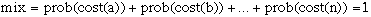where a, b to n are supporting strategies and prob(cost(a)),etc. is either the frequency of the strategy in the population orthe probability that a mixed strategist "adopts" that particularcost in a given contest.

Notice the last point -- as we learned earlier when we considered the"Hawks and Doves" game, there are two ways to produce an equilibrialmix. To this list, we'll add a third. A populationthat is evolutionarily stable could be:

• a population of pure strategists, each pure strategy is at its appropriate equilibrial frequency or
• a population of mixed strategists, each of whom can potentially play all strategies of the equilibrial mix at the appropriate frequencies. Thus, in a given contest a mixed strategist uses some mechanism to adopt a particular maximum acceptable cost at the correct frequency. What it adopts in one contest in no way influences what it will do the next time. -- or --
• a population that is a mix of supporting pure strategists (each at the appropriate equilibrial frequency) and mixed strategists (since they play each supporting cost at the equilibrial frequency). To take this a step further, the mixed strategists could even be "incomplete mixes" so long as they complemented each other and the net result was that in the population as a whole, the chance of any individual being in a contest with any strategy supporting the mix was always the equilibrial value for that strategy.

 This last point is very importantso let's make it one more time. All that matters for a populationto be evolutionarily stable is that: the fitnesses of each supporting strategy must be equal. As always, isofitness in no way requires that each supporting strategy actually has the same frequency! the mix is immune from invasion. It doesn't matter how the appropriate mix is obtained -- whetherit is from mixed strategy individuals, pure strategy individuals in thecorrect frequencies, or some combination of the two.

Return to the "Contents"

### Finding an Equation that Generatesthe Probability of Each Supporting Strategy at Equilibrium

As we start to look for a way to describe the mix, we seem to face adaunting task. We expect all possible costs to be members of this mix. Thus,there are an infinite number of supporting strategies each potentiallyat its own unique frequency.

So, we will not be able to use the simple technique to find the mix thatwe learned with Hawks and Doves. Instead of only needing a couple of linearequations to find two frequencies, we need a function that can give usthe correct frequency for an infinite number of different supporting strategies!What follows is a general description of the methods used by Maynard Smith(1974) to find this function.

 Please read this section carefully; it sets the foundation, establishesterminology, and reviews the mathematics used throughout the rest of ourtreatment of the war of attrition. Exposition that is not crucial (i.e.,can be taken on faith) is located on supplementary pages. Follow links tothese pages when you are confident of the basics -- they're worth lookingat, when you are ready.

Here we go. We shall use the payoff that a specific supporting strategyexpects to receive when competing against the 'mix' to find the functionthat gives us the equilibrial frequency of each strategy supporting themix.

So, we start with a pure strategy that is a member of the mix. (See aboveto review why a pure strategy can be part of the equilibrialmix.)

• This focal supporting strategy is willing to pay up to cost x=m
• So, we'll refer to it as fix(x=m)

Now, imagine that fix(x=m) is about to play a series of contests atrandom against other individuals (supporting strategies) from that mix.So, fix(x=m)'s opponent in any contest can be understood to be "mix"itself.

 Remember, it doesn't matter whether fix(x=m)'s opponent is a pure ormixed strategist: in either case we know the result is that onlyone strategy can be played by an opponent in a given game and the chancethat a particular strategy (maximum cost) will be faced is given by thecharacteristics of the equilibrium (review).

Let's find an equation for the payoff fix(x=m) receives againstany other supporting strategy in the mix, E(fix(x=m), mix). Starting,in general terms:

eq. #2: E(fix(x=m), mix) =

Lifetime Net Benefits to Focal Strategy in Wins

Minus

Lifetime Costs to Focal Strategy in Losses

 A reminder, gentle reader -- Remember our purpose in writingequations for lifetime net benefit and cost will be to extract a functionthat predicts the frequency of each component strategy of the mix.

In finding these equations, let's make one other important assumption-- we will assume that the resource has a constant value in any givencontest.

 Constant Resource Values? You may think that it is obvious that a resource value should be constantin any contest. There certainly are many if not most situations where thisis true. But, think for a moment and you'll realize that it is quite possiblefor a resource to become depleted during a contest. For example, individualsmay be contesting a resource that one of them already is using or that naturallydepletes in value over time independent of anything the contestants aredoing. Or, while two individuals contest for a resource, it is possiblethat another individual, perhaps a member of a different species depletesit. So, while reasonable for most situations, the assumption that for acontest V= constant may not always be justified.

Finding Expected Lifetime Net Benefits: Benefitsare only obtained by the focal strategist when she wins -- i.e.,when the focal strategist is willing to pay a higher cost than her opponentfrom the mix (x < m where m = the cost the focal strategistwill pay):

 eq. #3a: Net Benefit to fix(x=m) in a win = (V - x)

where V is the resource value and x is the cost the opponentfrom "mix" is willing to pay.

Unfortunately, equation #3a is not sufficient for our needs. Thecomplexity of the war of attrition intervenes!

Recall that the mix is composed of an infinite number of componentstrategies. Fix(x=m) only faces one of these supporting strategies inany given contest. Thus, equation #3a only describes the net gain in onespecific contest. You should realize that this particular contest will probablybe quite rare given the many different strategists that fix(x) could facefrom the mix. Thus, one particular contest and its benefits will have littleif any important lifetime effect on fix(x=m)'s fitness. Singlecontests cannot describe the net benefit that the focal supporting strategyexpects to gain from a large number (a lifetime) of contests.

To get an accurate measurement of lifetime net gains, we need to takeinto account all types (costs) of contests that fix(x=m) will win and theprobability of each:

 eq. 3b: Net Benefit = Sum{(V- x) * (Prob of facing x)}

(If you are having any trouble with this, please press here and read some more.)

Let's re-express eq. 3b using the notation of calculus (If youaren't familiar with calculus, don't fret because it will be fully explained!).We will use calculus because it will let us solve this complex problem (algebrajust won't work here) and because it will ultimately give us an exact answer.

First, the definitions of a number of symbols (most we have seen before):

• p(x) is the name of the function that can be used to find the probability that the opponent will play a given value of cost x

and to reiterate:

• V is the resource value; assumed to be constant in a given contest
• x: besides being a general symbol for any cost, x can also be used to indicate the maximum cost that some opponent from "mix" will pay. It has some value between zero and infinity, it is constant for a given contest, but it usually will be different in different contests.
• m is the specific maximum cost that our focal (fix(x=m)) contestant will pay, thus, m is a specific value of x.

Equationfor Net Benefit to Focal Supporting Strategy
vs.the Equilibrial Mix:

eq. #3c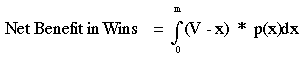Now, for those of you who haven't had calculus or who need a review,let's see what eq 3c means. First off, realize that it expresses the sameideas as does eq. 3b. With that assurance, let's start with the expressionto the right of the integration sign (the integration sign is theS-like symbol with m above and 0 below it -- moreabout it below):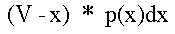This expression calculates the lifetime net benefit in winning a contestof a given cost x. Recall that V = resourcevalue and that x is the maximum cost that aparticular opponent is willing to pay. Thus, as in eq. 3a, (V - x) is the net gain to fix(x=m)(see below for note about wins). For example,if in a given contest V = 1.0 and x = 0.001 fitness units, the net gainin winning this contest is 0.999 fitness units. Note that we couldjust as well write(V-x) as (V-m) and we will later on.

Remember that it is not certain that fix(x=m) will play any particularsupporting strategy in the mix. Instead, the probability of playing againsta particular strategy x supporting the mix is p(x)dx wherep(x) is the function that we want to discover to complete the descriptionof the mix. The notation dx that follows p(x) simply meansthat we will multiply p(x) times an infinitesimally small value of cost.So, solving p(x)dx will give us the chance that our focal fix(x=m)strategist faces any particular value of x from the mix.Be careful notto assume this means some variable "d" times the cost x that"mix"adopted in this game. Also, don't make the common mistake of thinking thatdx increases as x increases. It is a constant, tiny amount of cost.

Finally, there is the integration sign: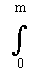Specifically, thisis a definite integral. It says to add up all values of (V-x)*p(x)dxbetween costs of x = 0 (the number underneath the integration sign)up to x = m (above the integration sign). (Note -- it is a definiteintegral because these limits are given -- when limits are not given (indefiniteintegral) we integrate over all possible numbers. However, since costs canonly be positive or equal zero, we need to use this definite integral!).

Notice how the limits of the integration arecrucial for defining what is a victory by fix(x=m) over mix. As longas the x from the opposing "mix" is less than m, then fix(x=m)wins and the expression calculates the added lifetime net benefit of thiswin.

To summarize: for any contest where x < m, we

• perform the operation (V-x)*p(x)dx and
• add the result to all other cases where x < m.
• When we have completed this, we have the expected lifetime net benefit that fix(x=m) should accrue in contests it wins.

 To make this concrete let's use the followingvery inaccurate example (more about why this is inaccurate later). Assume V=1 and that fix(x=m=0.21). Further assume that only values of mix are x=0 (at a prob of 0.3), x=0.1 (at a prob. = 0.2) and x = 0.2 played at a prob = 0.1. (Using such a small number of widely dispersed values is where much of the inaccuracy of this sample calculation enters.) Then we will "integrate" between 0 and m= 0.21.At x = 0, the net benefit is (1 - 0)*0.3 = 0.3.At x = 0.1, the net benefit is (1 - 0.1)*0.2 = 0.9 * 0.2 = 0.18At x = 0.2, the net benefit is (1 - 0.2)*0.1 = 0.8 * 0.1 = 0.08The sum of all of these between 0 and m is 0.3 + 0.18 + 0.08= 0.56 -- the expected net gain for fix(x= 0.21) in wins against membersof our unrealistic mix!

 Note: Kevin Mitchell of Hobart and William Smith Colleges hasprovided an excellent overviewof integration and probability density functions as a PDF document.It is a bit more elegant than the treatment given above!

Expected Lifetime Costs for Losses: Benefitswere the hard part of the E(focal supporting strategist, mix) equation.Calculation of lifetime costs to focal strat fix(x=m) in contests itloses to the mix (i.e., a mix strategy opponent) is much easier.

As before, the logic is simple. Fix(x=m) loses whenever x, the cost theopponent from mix in any particular contest is willing to pay, is greaterthan m. All of these contests end with a cost = m. Therefore, forany one losing contest:

 eq. 4a: Cost to fix(x=m) of Loss= (- m)

So, unlike the equation for net benefit, the costs in any loss are alwaysthe same. But, we're not done because as with net benefits, we need to takeinto account the proportion of the time fix(x=m) encounters an opponentthat (in this case) it loses to:

Lifetime Costs of Losing to the Mix
(i.e., Losing to a Mixed Strategist)

eq. #4b: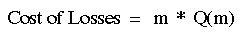where m is the maximum cost that our focal supporting strategywill pay and the function Q(m) gives the lifetime proportion of timesthat fix(x=m) loses to another member of the mix. Now: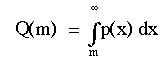Once again, some explanation of this equation:

• to find Q(m) we take the definite integral of the probability of facing each specific opponent (cost), given as p(x)dx
• we do this between between m (the first contest cost where our focal strategist starts to lose) and infinity (the most costly possible contest)
• m stands for any cost
• This gives us the total chance that our focal strategist will lose to the mix, i.e., Q(m).

To recapitulate, to get lifetime expected cost of losses, we simply multiplythis cost times the chance that fix(x=m) will lose.

Notice that as with net benefits, the function p(x) is central.

 Continuing our example from the last box:Recall our focal supporting strategy Fix(x= m = 0.21).From the last box, we know that contests where fix(x=m=0.21) won madeup 0.6 of the total contests (we get this by summing of the probabilityfor each winning contest -- 0.3 + 0.2 +0.1 -- a "poor mans" integration). Thus, the chance of not winning is 1.0 - chance of winning = 1 - 0.6 = 0.4. Therefore the cost of losses was 0.4 * 0.21 = 0.084 fitness units.

So, to get the expected lifetime payoff to fix(x=m)vs. the equilibrial mix, we simply substitute the two equations fornet benefit and cost:

eq. #5: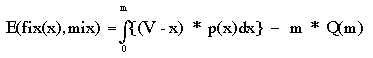Completing our far oversimplified example, the result is:

E(fix(x=m), mix) = 0.56 - 0.084 = 0.476

 Important Note: we will see in our "grand review"at the end of this page that E(fix(x=m), mix) actually always equals0 in the mixed ESS for the war of attrition! Again, please excuse my use of an inaccurate example; it was doneonly to help you understand the calculations, especially if you haven'thad calculus.

Now we have the payoff equation (eq. #5 ) that contains the function p(x). How doesone solve to find the function p(x)? It is not terribly difficultbut then neither is it central to our story. At some point, if you are interested,you should take a look. But for the moment, we'll proceed directly to thenext section where we'll introduce the result that Maynard Smith obtainedfor p(x) and we'll discuss it in considerable detail.

Return to the "Contents"

### The Mathematics ofthe Mixed Equilibrium in the War ofAttrition

Recall that Maynard Smith's goal was to find a function, p(x),that would supply the frequencies of each supporting strategy (cost, x) for an equilibriumin the war of attrition. To get p(x) he solved eq. 5 and obtained the following result:

 eq. #6: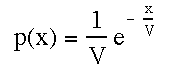where p(x) is the probability densityfunction (dimensions of probability per unit cost), xis cost, V is resource value ande is the base of the system of natural logarithms(e about equals +2.713).We will also write this expression as 1/V*exp(-x/V) where exp(-x/V) isthe same thing as writing e to the negative (x/V).Important note: Remember that exp(-x/V) is the equivalent of 1/exp(x/V).Negative exponents are the same thing as the inverse of the expression.So 2^ -2 = 1/2^2 = 1/4!

Eq. #6 is an example of type of function called a probabilitydensity function.

 Negative exponential distributions are an example of a very importantgroup of functions called Poisson distributions. Press hereto read a bit more about Poisson distributions

However, it does not give frequencies of different maximum acceptablecosts. Instead, true to its name, it gives probability density: probabilityper unit x. To make this a bit more concrete, solutions to eq. #6 giveprobability (or frequency) per unit cost.

 Details About Probability Density Functions:Now that you are somewhat familiar with the probability density function,p(x) you may wish to learn about this type of function in more detail. Followthe link below to read about: the differences between probability density and probability (which cause most students considerable confusion), the differences between continuous and discrete variables, why the chance of a particular value with a continuous variable is usually vanishingly small and how to use eq.6 to find probability.Note -- if you are still a bit shaky on the math, read the restof this section to get an overview and then visit the Probility Densitylink.

Another Note -- Probabilities and Frequencies: I was not pullinga fast one when I equated probabilities and frequencies. A quick review-- remember that a frequency is simply the proportion of the total madeup by one particular class. For example, if 20 out of 1000 in a war of attritionwill pay a cost of up to 0.08 fitness units, the frequency of individualspaying a maximum cost of 0.08 is 0.02 . By the same token, if we were torandomly pick an individual from this population, the chance of pickingan individual who would pay a maximum of 0.08 would be 0.02 (2%). The maindifference in common usage between the terms probability and frequency isthat probabilities are usually theoretically expected proportions whilefrequencies are often actual measured values. However, probability valuesare often used synonymously with expected frequencies in theoretical distributions;that is what we will be doing for the rest of this section.

How do we get simple probability (frequency)? We need to multiplyp(x) by cost. Now the earlier equations that contained p(x) (e.g.,eq. #5)should make a bit more sense. Notice that they contained the expressionp(x)dx which means to:

• find the probability density associated with some value of cost x
• multiply that result times an infinitesimally small increment in cost
• the result is the frequency of individuals willing to play (pay costs) up to that particular exact value of x.

A word about probabilities and ranges of cost. Since cost is a continuous variable,for any exact value of cost the frequency of contestants who play that exactvalue is exceptionally low (unless we are dealing with the exceptional caseof p(x)d(x)=1.0 -- also see the grey box above) .Probability accumulates as a continuous variable changes. Thus, thegreater the range of the costs that we consider, the greater thefrequency of individuals between those costs (alternately, the greater theprobability that a mixed strategist will quit between these two costs ina given contest).

As you probably (no pun) know, integration would be the best techniqueto apply to the problem of finding the frequency of individuals willingto pay or not pay a certain cost x. Recall that when we integrate, we invokeproven mathematical techniques that have the effect of adding togetherthe results of solving for p(x)dx at each x(each tiny step). (Actually,the way I just described the process is a bit more like the way a computerwould accomplish this operation, but in any case, it gives you the rightidea about what integration accomplishes.) Thus:

eq. #7: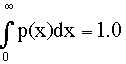where p(x) is the probability density function(dimensions of probability per unit cost) and dx is a tiny incrementof cost.

What eq. #7 says to do is:

• for each tiny increment in cost dx from zero to infinity (notice that we are sequentially dealing with every possible cost x).
• solve p(x). Since we are proceeding in infinitesimally small steps (dx) from zero to infinity, note that in effect we will perform this calculation for every value of cost between and zero and infinity.
• multiply the result of solving p(x) for each cost times the tiny cost increment dx (note -- times the increment, not times the actual cost)
• add all of these results together
• Since in this case we calculate the probability of playing all possible costs, then the sum of all of these probabilities must be 1.0.

 What was just described is functionally what happens when we solve eq. #7.But in some ways it more closely resembles the way that a computer wouldsolve the problem. We don't actually solve the equation using the stepsexactly as outlined. What happens with a calculus solution is that we applycertain rules to give us a solution to eq. #7 that has the effect of thesteps mentioned above.

To gain a bit more understanding, let's see an example. Let's solve eq.#7 using the rules of integral calculus (if you've taken calculus this willbe familiar, if not, just realize that we apply some rules to get the expectedresult)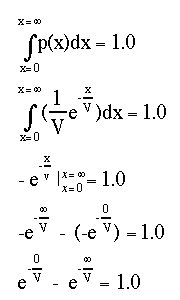Here's a step by step analysis:

• the top expression is eq. #7
• the next is eq. #7 with eq. #6 substituted for p(x)
• the next two steps involve the calculus; let's not get into it here except to realize that the transformations that occur here are the equivalent of all of the steps mentioned above
• Important note: Remember that exp(-x/V) is the equivalent of 1/exp(x/V).

Now since:

• any number raised to the 0.0 power equals 1.0 (remember 0/V is still 0) and since
• any number raised to negative infinity approximates zero (remember that infinity/V still equals infinity -- try a very large negative number on a calculator if you don't believe it), then:

 1.0 - 0.0 = 1.0

Now you should have the basic idea about how we go from the probabilitydensity function (eq. #6) to probability. Since we're on the subject, let'ssee how we calculate the cumulative (total) probabilities (frequencies)of playing up to or beyond any particular cost (we alluded to thesecalculations earlier when we wrote expressions for net benefit to a supportingstrategist (eq.3c and 4)). Let's also see how to integrate eq. 6 to get an expressionthat tell us the chance that an individual plays up to a certain time.

First, let's find an expression for the totalproportion of individuals in the mix who are expected to have quit betweencosts between zero and cost x=m (this of course is the same as givingthe chance that a mixed strategist will quit by cost m). This is calledthe cumulative probability distribution of quitting times, P(m)

eq. #8a: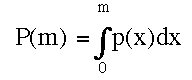Let's discuss this equation.
P(m) is thedefinite integral of the density function p(x) between costs between0 and m. Note that in eq. 7 we considered the inclusive range of costs between0 and infinity. So the only difference here is that for each contest costx, solving for P(m) will give us the chance that an individual has quitbetween the start of the contest and any cost m. Alternately, it would giveus the percentage of a population that has quit as of a certain cost. Itdoes not give us the chance that an individual will quit at some small specificrange of costs (see eq. 10 for that).

Upon integrating eq. 8a we get a formula from which we can readily calculateP(m) for any particular cost (x=m):

eq. #8b: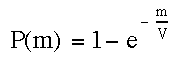To reiterate: when we solve eq. 8b for any cost, the result willbe the total proportion of a population of mixed strategists who would havequit as of cost m. Again, remember that this does not mean that they allquit at cost m. Instead, P(m) includesthose quitting at cost m AND all that have quit before cost m. Hereare plots of P(m) for three resource values (V) over a range of costs betweenx = 0 and x = 10: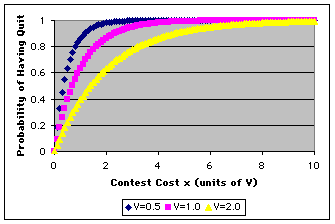Notice that in all cases the initial chance of having quit is (of course)zero. As contest costs accumulate, it becomes more likely that one willhave quit since costs start to exceed the maximum different supporting strategiesare willing to pay. (Note: we have talked about individuals who quitat cost = 0; assume that what really happens is that they quit after a smallcost, 0 + dx, is paid).

Another way to think about these plots is to imagine 1000 identical'mix' strategists starting a display game. At time zero, all are playingso zero have quit. A short time later some have quit, as time goes on agreater and greater proportion have quit and so the overall chance thatan individual who started the game will have quit gradually increases.

The other thing to note is the effect of V on quitting. As V getslarger, individuals quit at lesser rate (fewer quit per increase in costx). This should make sense -- a contestant should be less likely to giveup over a valuable resource. In fact, the rate of quitting is proportionalto 1/V; more about this below.

 Exercise: Before going any further,be sure that you can solve the cumulative probability distribution equationP(m). To solve this problem, you will need a calculator or spreadsheet withnatural logs (exponentiation of e, often called exp). Alternately,if you can use the number 2.72 whenever you need e.1. What is the cumulative chance of quitting between a cost of 0 andinfinity if V=1? V=5? V=0.5? Ans2. What is the cumulative chance of quitting between a cost of 0 and0.6 if V=1? V=0.5? Ans.

Hopefully this is all starting to make a lot of sense. Now let's lookat the converse of the cumulative probability of having quit as cost x=m(alternately -- the total frequency of quitters as of cost x=m). The conversewould be the cumulative frequency who have not quit as of cost m(a.k.a. "probability of not having quit", or the probabilityof enduring to a certain cost); we call this Q(m) and we sawit earlier with the equation for net cost to any supporting strategy vs."mix":

OK, if P(m) is the cumulative chance that an individualwill have quit as some cost, then 1- P(m) will be the chance that theyare still playing. We'll call this Q(m): the probability ofenduring up (not having quit) to a certain cost. Here is a graph forQ(m) when V=1:

 eq. #9: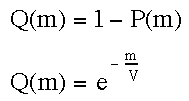We can of course find Q(m) by integrating p(x) from m to infinity.Press here if youwant to see this integration.Now, as with P(m), if we solve eq. #9 for a series of values ofcosts we can get a plot of the cumulative chance of enduring (not quit)as of any cost m. Review the plotfor P(m) and then try to imagine how this graph should look. Afteryou have thought about this, press here to see the plot of Q(m) vs. cost.

Notice that eqs. 8 and 9 both give us cumulative probabilities.This means that both give frequencies/probabilities starting at zeroup to some cost x=m (thus, if that cost x is infinity, then the cumulativechance of having quit by that cost is 1.0 and the cumulative chance of nothaving quit is 0).

But what if we simply want to know the chancethat an individual will quit over some specific cost range -- for example,between cost x1 = 0.50000 and cost x2 = 0.50001. This is especially usefulin understanding how a computer solves the war of attrition such as inthe war of attrition simulation that accompanies this page.

• All we need to do is subtract the cumulative (P(m) or Q(m)) values for two different costs. So we will call this probability deltaP(m) or P(m1<=m<=m2) -- this second statement says "the probability of quitting associated with selecting a value of m within the specific interval m1 to m2".
• We can also get deltaP(m) by simply integrating between any two limits instead of between the specific cost = 0 and any other value of cost. Here is that solution

 eq. #10: Calculation of deltaP(x)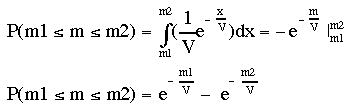notice that this is the same expression as: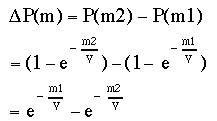So, we have now gone over the equations that can give us various probabilitiesor frequency distributions in the war of attrition. All of these are the"children" of eq. 6, the probability density function that Maynard Smithderived to describe the mixed ESS. We will use these functions in the discussionsthat come below or on related pages (for instance, we will use eq. 10 on a related page that considers how a computer wouldsolve the war of attrition).

In the next section, we will talk about what eq. 6 really means: whatdoes it say about mixed strategies in the war of attrition. After we havea full description of this mix, we will turn ourselves to our final task-- proving that the mix is an ESS.

QuestionsAbout Chances of Continuing

1. Name the probability distributionsthat we saw earlier that give (i) chances of continuing to a certain costor (ii) quitting as of a certain cost. Answer

2. If eq. 11 gives the chance of continuing for a unit ofcost, write an expression that gives the chance of quitting per unit cost.Answer

 If you need more review about the influences of V andx on the chance of continuing, press here to see some additional explanation

Return to the "Contents"

### Getting it Together: A Descriptionof the MixedEquilibrium in the War of Attrition

We are now at a point where we can understand the characteristics ofthe mixed equilibrium. As mentioned previously, this equilibrium could consistof either:

• a mix of individuals who played different pure strategists (single maximum costs) but where the frequency of each pure strategy type was equilibrial (as ultimately described by eq. #6), OR,
• a population consisting entirely of mixed strategists -- that is, individuals who were capable of playing any strategy in a given contest so long as the probability of playing a particular maximum cost was ultimately given by eq. #6, OR,
• some mix of the two above, including perhaps alternative versions of mixed strategists so long as the overall frequency of each supporting strategy in the population as a whole was in line with eq. #6.

Thus, eq.#6 has a key role in describing the equilibrium.

In this section we will focus on the characteristics of the equilibrium.How should members of a population at this equilibrium act?

 Important ConventionFor convenience we are going to think about our population in terms ofthe second possibility just discussed -- we will regard the equilibrialpopulation as consisting entirely of mixed strategists, all of whom arecapable of playing any maximal cost with a probability ultimately describedby eq. 6.

Since other mixes are possible we'll give this particular mix a name'var' for variable cost strategist.

 A Note About Strategy NamesUsed on the Remainder of this PageSome of this is reiteration of what was just said but please glance overit so that you are familiar with the strategy names and definitions we willuse from here on out.The names and symbols we will use for the strategies are a bit differentthan those used by Maynard Smithand Bishop and Cannings.They are meant to be more descriptive and therefore easier for someone toremember; hopefully this use will not result in any confusion. to thosefamiliar with these author's work. I do this with some reluctance buthave found that my students seem to have an easier time this way as comparedto using symbols such as I and J or the generic term"mix". So: As just mentioned, we'll call the evolutionarily stable mix discovered by Maynard Smith 'var' for variable display cost. Var consists of all possible costs played at frequencies determined by the probability density function, eq. 6. Var will be the center of most of our discussion on the rest of this page. The term "mix" will apply to any mixed strategy -- i.e., a strategy that conforms to eq. 1. the name "fix(x)" will apply to any pure strategy whose players select a fixed maximum display cost x (=time). Thus, there are potentially an infinite number of versions of fix(x) each characterized by different maximum costs but all sharing the characteristic that over a lifetime they have but one maximum cost (in contrast to var). We have previously considered fix(x) in detail (press here to review the page describing fix(x) strategies and why they are not evolutionarily stable). For the rest of our treatment of the war of attrition, we will regard fix(x) strategists not as supporters of the 'var' equilibrium but instead as competitors, i.e., potential invaders. Just think of them as attempting to invade a population consisting entirely of mixed strategists; the addition of any fix(x) strategist will have the effect of changing the frequency of a particular maximum acceptable cost (which can be generated by either a var strategist or this fix(x) invader) from the equilibrial value given by eq. 6. We're going to learn whether or not this alteration will be permanent.

What are the characteristics of ourmixed strategy "var"?

1. Like other strategies, 'Var' is highly secretive! There can NO INFORMATION TRANSFERfrom var to its opponent THAT MIGHT SIGNAL WHEN 'VAR' WILL QUIT.

• Thus, the opponent of a var strategist never knows nor never can know exactly when the var strategist will quit. No factor (e.g., physiological condition or some intention movement) can be allowed that might tip off the opponent as to var's intentions.
• Obviously, if such information transfer occurred, it would be easy to create a strategy against var (out-wait var in any contest up to m>V/2, quit at m=V/2).
• This is one of the few important characteristics of 'var' that is not subsumed by eq. #6. But note that it is also a characteristic that any strategy should possess. For instance, if a fix(x) strategist tips its hand, it would also place it at a disadvantage.

2. Varstrategists may potentially play any cost -- from no cost to (theoretically)an infinite cost. We discussed the reasons for this in the first sectionof this page (review).

3. 'Var' strategists have a constant rate of continuingover each unit of cost. The chance of continuing is proportionalto 1/V; this quantity is also known as the rate constant (presshere if you want to reada bit more about rate constants). The chance of continuing perunit cost:

 eq. 11:Prob. Continue PerUnit Cost x = exp(-1/V) = 1 / exp(1/V)(note that this equation is the same as eq. 9 when Q(m) is solved for x= m = 1)

Thus, with regard to the chance of var's continuing to display:

• the exponent of e in eqs. 6, 8, 9 , and 10 , x/V, is nothing more than a Cost/Benefit ratio -- (i.e., the greater the chance of quitting)! Looking at cost and benefits separately shows is also instructive:
• the larger this C/B, the smaller the chance of continuing
• so, since the chance of quitting is the inverse of continuing, the larger the C/B, the greater the chance of quitting
• Thus:
• the chance of continuing is DIRECTLY proportional to the resource V. This should make good intuitive sense -- the more valuable the resource the less likely a contestant should be to quit in a given increment of cost.
• The chance of continuing is INVERSELY proportional to the cost or cost increment -- the greater the cost, the lower the probability of continuing.

 If you don't spend a lot of time dealing with exponents, theselast two statements might confuse you. It is very important that you keepin mind the fact that x/V is part of a negative exponent.Thus: If cost (x) gets larger 1/exp(x/V) gets smaller. On the other hand, if V increases, 1/exp(x/V) gets larger. So, the usual rules about a numbers in a fractional exponent have been reversed . To reiterate -- if the exponent is negative: an increase in the numerator of the exponent means that result is smaller (the numerator and the result are inversely proportional to each other); if the denominator of a negative exponent increases, the result increases (the denominator and result are directly proportional to each other).

4. Now, since the behavior of a 'var' strategist is determinedby a certain chance of quitting with each unit of cost, and since varnever tips its hand, you should realize that an opponent will never knowexactly when a 'var' strategist will quit -- anymore than you, me oranyone can always correctly guess when a "fair" coin will turnup "heads". Thus, knowing when something will happen is quitedifferent from knowing the chance of some event. This is the essence ofthe problem var's opponents face!

5. Another result of a constant chance of continuing per unitcost (i.e., a constant chance of quitting per cost) is that thechance of accepting greater costs (i.e., of playing from the start throughto cost x) decreases exponentially (for any value of V less than infinity,i.e., for any exp(-1/V) < 1.0). The effect of this is that there is virtuallyno chance that a var strategist will be willing to pay a cost thatis very large compared to V.

• What this means is that even though the chance of remaining or quitting is always the same for those who are still playing the game, the number of players will drop most rapidly at the start and then more gradually as the number of players approach zero.
• We have already seen this in the plot of Q(m) (the chance of playing from the start to a particular cost m) vs. cost.
• As mentioned earlier, we call this type of plot an exponential decay. Examples of exponential decay include eqs. 6 (prob. density), 9 (Q(m)), and 10 (deltaP(m))

6. To summarize, the opponent:

• can have a general idea of what a var strategist will do in a contest for a given resource if it has a knowledge of the distribution of 'var' strategists willing to pay different maximum costs, Q(m)

• However, the opponent can never consistently predict var's actions in any particular contest. That is because 'var's actions at any cost are totally independent of anything that it did in previous games -- whether it continues from one moment to the next is simply a matter of a constant chance factor.
• Thus, "'var' is predictably unpredictable".

 Link to an Illustration of "Var - Like" BehaviorThe last statement is perhaps the most crucial in understanding the behaviorof 'var' strategists. Central to it are the ideas of constant probabilityof continuing the game and independence of decisions from one moment (cost)to the next. You will also explore this in great detail when you run thesimulations. For the moment, however, take the time to read an example illustrating how a strategy like 'var' works.

 Questions About the MixedStrategy Var1. Compare what a contestant sees when itconfronts a population consisting entirely of 'var' strategists as comparedto a population that is an equilibrial mix of pure supporting fix(x) strategies.Would the contestant see any difference in these two situations? Answer2. How would you express the idea of constantrate of quitting with respect to a population of pure strategists who togetherproduce an equilibrium? Answer3. Why is it crucial that no informationas to var's intention to continue or quit a contest be passed onto its opponent? Answer4. How do you estimate the probabilitythat a var strategist will win a contest of cost x? Answer5. How do you estimate the probabilitythat a var strategist will lose a contest of cost x? Answer6. How do you estimate the probabilitythat a var strategist loses by paying a cost between x and x+dx?AnswerAll of the remaining questions call for solutions to equations derivedfrom eq. 6, the probability density function that describes var. You willneed a calculator or spreadsheet with natural logs. Alternatively, you canuse the number 2.72 whenever you need e.7. Should the chance of encounteringa member of the "stable mix" with a quitting cost between 0.60and 0.61 be greater or less than encountering an individual with a quittingcost between 0.60 and 0.62? Explain. Answer8a. What is the chance of encounteringa member of the stable mix with a quitting time between a cost of 0.60 and0.61 if V=1? V=0.5? Compare these answers with the next question. Answer8b. What is the the chance of encounteringa member of the mix who quits between a cost of 1.0 and 1.01 if V=1? V=0.5?Compare these answers with the last answers. Why the difference? -- thesize of the cost interval is the same Answer.

Return to the "Contents"

### Proving that 'Var' is EvolutionarilyStableA. Requirements of Proof

We now know the general characteristics of the mixed strategy we call'var' -- the rangeof its maximum display costs, the probability of playing each of these costs,and the relationship of these probabilities to the resource. And we knowthat the equation that eq. #6, which describes var's behavior sprung from theassumption that:

 E(any fix, var) = E(any mix, var) = E(var, var) = constant

Finally, we know that Bishop and Cannings (1978) have showed that thisassumption must correct for any ESS in the symmetrical war of attrition(see Bishop-Cannings theorem).

However, simply showing that the 'var' strategy has some behavior consistentwith being an ESS is not the same thing as showing that it is an ESS. Recallthe two general rules for finding ESSs we learned about earlier .'Var' is an ESS (cannot be invaded if sufficiently common) if:

 Rule 1(common interactions): E(var, var) > E(fix(x),var)(the equilibrium property)ORRule 2:IF (common interactions):(part a):E(fix(x),var) = E(var, var) THEN (rare interactions):(part b): E(var,fix(x)) > E(fix(x),fix(x))(the stability property)

Now, in the case of 'var' we are only interested in rule #2 sincewe already know that part a of rule #2 is true. In fact,'Var' isderived from part a! And of course rule #2 is not consistent with rule #1.But just because 'var' is derived from rule #2(a) does not mean thatit must be consistent with rule #2(b). And if 'var' vs. any fix(x)is not consistent with part B, then var is not an ESS (see box below).

 If 'Var' Were Not an ESS, What Would It Be?If 'var ' vs. any fix(x) is only consistent with rule 2 part A, it isequilibrial. This is because if E(var,fix(x)) > E(fix(x),fix(x))is false, then the only interpretation that is also consistent with rule2A is that E(var,fix(x)) = E(fix(x),fix(x)). So, the common interactionswould have the same fitness consequences on each party (no advantage toeither) and the rare interactions would also give no advantage to eitherstrategy. Note that the payoffs in common vs. rare interactions would nothave to equal each other, the only equality needed is that common are equalfor both as are rare. The result is that selection could not change thestrategy frequencies and we would say that the population was equilibrial.(The only way that frequencies can change are by mutation, immigration oremigration.)

So, to show that 'var' is an ESS all we need to do is to show thatrule #2 part b holds:

 Rule 2, part b: E(mix,fix(x)) > E(fix(x),fix(x))

What will follow is a mathematical proof that rule 2b is in fact trueand therefore that 'var' is an ESS in the war of attrition. Once again,there will be a bit of calculus to enhance the argument but anyone shouldat least be able to follow the outline of the proof. As before the calculusis all explained, furthermore, much of it is very similar to what we haveseen earlier. And, to make the concepts clearer, a number of graphs willbe presented.

Return to the "Contents"

### Proving that 'Var' is EvolutionarilyStableB. The Proof

Once again,'var' is an ESS if:

 Rule 2, part b: E(var,fix(x)) > E(fix(x),fix(x))

is true.

So, we will need to find expressions for E(var,fix(x)) and E(fix(x),fix(x))and determine whether or not the difference between the two is always apositive number -- i.e.,

 eq. 12: E(mix,fix(x)) - E(fix(x),fix(x)) > 0

Now, recall eq. #2 from earlier. The payoff to a given strategy in acertain type of contest is always:

 eq. #2: E(focal strat., opponent) =Lifetime Net Benefits to Focal Strategy in WinsMinusLifetime Costs to Focal Strategyin Losses

So, let's find the net benefit and cost equations for E(mix,fix(x))and E(fix(x),fix(x)) and then substitute them into eq. 2 before finallysolving to see if we have an ESS. We'll use the same general symbolsand operations that we used in finding E(fix(x), mix (i.e., 'var')) earlier.

Part One: Calculation ofNet Benefits

The benefits needed to calculate these payoffs are easy to find and sothey represent a good place for us to start. First, recall that we assumethat the value of the resource is constant in any given contest; furtherwe assume that it has the same value to both contestants. As usual,we will symbolize it as V. Here are the net benefits for each typeof interaction.

Net Benefits to Var in Contests vs.Fix(x): Remember that var does not enter a contest possessinga particular maximum cost that it is willing to pay. Instead, at eachinstant it has a constant probability of quitting proportional to 1/V.Thus, it is unpredictable as to exactly when it will quit.

Now remember that in wars of attrition, winners, like losers, paycosts. These costs lower the net (realized) value of the resource tothe winner (press here to reviewour assumptions about costs):. We'll call the maximum cost the fix(x)strategist is willing to pay m). So, against a given fix(x=m)strategist, 'var' wins whenever it is willing to pay more (i.e.,whenever it continues to play after fix(x=m) quits). Thus, when 'var'wins, it will always win V-m. But it is not certain that 'var' will playto a higher (winning) cost than fix(x=m) since var uses a probability functionto determine when to quit. So, 'var' expects to get:

 eq. 3b: net Benefit = (V - m) * (Chance of winning)

Recall from earlier that the chance that 'var' has not quit as ofpaying any cost x= m is Q(m):

 eq. 8 and 13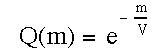Recall that this equation finds the chance that var has not quit asof cost m by adding up all of the probabilities of 'var' quitting at costsgreater m.

So, after substituting eq. 13 into the net benefit equation (#3b), thebenefit to 'var' is:

eq. #14a: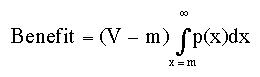Notes about the equation: Notice that (V-m) is placed outsideof the integration sign. That is because in the case of 'var' against agiven fix(x=m), 'var' can never expect to win anything except V-m. So, (V-m)is a constant for a contest that can last up to any given cost m. And 'var'only wins when it has not quit as of m. And, of course, the purpose of theintegration is simply to find the chance that var will still be playingas of cost x=m.

### Solving eq. 14a: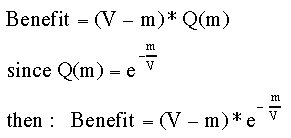Net Benefits in Fix(x)vs. Fix(x) Contests: In this contest we have two identical fix(x)strategists facing each other. Thus, they play to exactly the same costx=m. Since we assume no other asymmetries, then it is bestto assume that two identical individuals will each win 50% of the time-- they will in effect split the net benefits. Thus:

 eq. 15: B for fix(x) vs.fix(x) =0.5 * (V - m)=0.5 * (V - x)

Part Two: Calculation of the Costof Losing

Calculation of Cost to Var Strategists in Lossesto Fix(x): The calculations for lifetime loss costs to 'var' are a bitmore complicated than those for net benefit. The reason is that 'var'can lose to a given fix(x=m) many ways!
Here's an example.

• Suppose that a 'var' strategist repeatedly plays a fix(x=m=1) strategist in contests where V=1. What happens in terms of costs?
• We know that 'var' loses anytime it quits before paying a cost slightly greater than 1.
• There are many ways that a 'var' strategist can lose to a fix(x=1) strategist over a repeated number of games because 'var' can play a potentially infinite number of losing costs (i.e., costs between 0 and 1) against fix(x=1).
• Each of these losing gambits (costs) has distinct probability of occuring.
• So, over a lifetime, the cost that a 'var' strategist expects to pay when it loses to a given fix(x=m) will be equal to the sum of the product of each unique losing cost and the probability of playing that losing cost.

Let's express this idea mathematically:

 eq. 16a:Let's be sure we understand what eq. 16a means: x is the cost 'var' paid as of the moment of quitting, and p(x)dx is the chance of quitting between cost x and the next infinitesimally small increment in cost. Thus: the product of the two (x and p(x)dx) is the expected lifetime cost to var of playing to a particular cost x and then quitting. Now, since there are many ways to lose, therefore we must sum (integrate) the values expected for each contest cost (x*p(x)dx) between x=0 and x= cost the fix(x=m) opponent is willing to pay. This sum is the the lifetime cost 'var' expects to pay in losing contests where the opponent is willing to pay a certain amount m.

We can solve eq. 16a by inserting eq. 6 for p(x) and integrating:

 eq. 16b: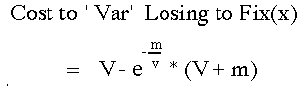If you understand calculus and/or if you are sure that you understandhow costs are calculated, you can move on to the next section. If not, pleasevisit the following link which will take you to a discrete calculation of net benefits and costs.

Calculation of Cost to Fix(x) Strategists When vs. Fix(x): Onceagain, this is a very easy calculation. The contestants are identical --both are willing to pay cost x=m. As we said in our considerationof benefits, we simply assume that each individual wins 0.5 (50%) times.So, half the time they lost and pay cost x=m:

 eq. #17: Cost paid by a fix(x) in losingto a fix(x):= 0.5 * x = 0.5 * m

Part Three: Payoff Equations

Section A: E(fix(x=m), fix(x=m)):Let's start with fix(x) contests that end in ties (since they're easy).Now, since

 eq. #2: Payoff(to Strat., when vs. a Strat.) =(Benefit from win) - (Cost from loss)if we simply substitute equations for benefit in winning (eq. 15) andcost in losing (eq. 17) we obtain:eq. 18 E(fix(x=m), fix(x=m)) = 0.5*V - m

Section B: E(var, fix(x=m)): Thistime we substitute eqs. 14 and 16 into eq. #2 :

 eq. 19a: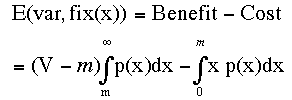and if we integrate this equation we obtain the following result:eq. 19b: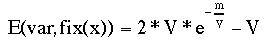(You have seen the steps to this integration previouslywhen we considered cost and benefits, but you may press hereto review those steps)

 At this point you can either continue on to the final proof that'var' is an ESS or you might find this a good place to take a side tripthat explores the differences between the 'var' and fix(x) strategies bypresenting graphs of benefits, costs and payoffs for each strategy.Presshere to go to a graphical presentation of benefits, costsand payoffs in 'var' and fix(x)

### The Grand Finale: The Mixed Strategy'Var' is Evolutionarily Stable: Showing that E(var,fix(x)) > E(fix(x),fix(x))

Recall from above that to prove that 'var' is evolutionarily stable thatwe need to show that rule 2b is correct. Here we go:

 Finding an Equation for the Difference in Payoffs Starting with rule 2b:E(var, fix(x)) > E(fix(x), fix(x))and rearranging, we get:E(var, fix(x)) - E(fix(x), fix(x)) > 0Now since:E(fix(x) ,fix(x)) = 0.5 V - m (review)and sinceE(var, fix(x)) = 2 * V* exp(-x/V) - V (eq. 19b)then:2 * V* exp(-x/V) - V - 0.5*V - m > 0which simplifies to:eq. 20: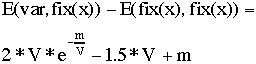Now the big question -- is eq. 20 always positive as it must beif 'var' is an ESS?

We could start out by simply graphing it. If we do so for V=1we will see that there is no place where E(var,fix(x)) < = E(fix(x),fix(x)):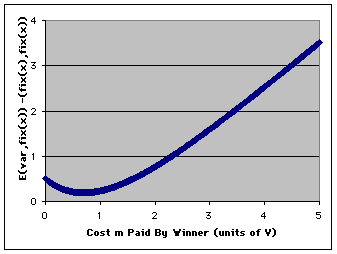(Looks like the "swoosh" doesn't it!).

Thus, it would appear that 'var' is stable. But not so fast -- this isfor only one value of V. Is it possible that there are values of V where'var' is not evolutionarily stable? After all, V does affect 'var's behavior.

As with finding the frequency of each maximum acceptable cost (when welooked for p(x)), solving for every possible V might appear to be a difficultproblem (and approached that way, it is!). However, once again a bit ofelementary calculus can come to our aid and comfort.

Mathematical Proof: To show that no pointon eq. 20 is less than or equal to zero, we need to find the minimumvalue of eq. 20. This occurs where the slope of the graph is zero(the flat part of the graph above; on that graph it happens at a value somewherenear cost = 0.7).

• To find this point for any V, we use the calculus technique of differentiation. It will give us an equation for the slopes between every two adjacent points of a plot of eq. 20.
• If we then solve this "equation of slopes" for the cost where the slope equals zero we find that this always occurs at 0.693 * V.
• Now, all that remains to do is to substitute this value (0.693V) back into equation 20 and solve for E(fix(x=m)- fix(x=m)). The result is the minimum difference is always +0.193*V.

Thus, 'var' is an ESS!

 Presshere to see the mathematical details of finding the minimum difference betweenE(var,fix(x) and E(fix(x), fix(x))

Graphical Illustration of the Proof: Ifyou are not fully confident that you understand the proof, you will probablybe reassured if you look at the graphs below of eq. 20 for different valuesof V. Remember, we have said that the minimum difference in fitness willalways = 0.193*V and will always occur at cost = 0.693*V: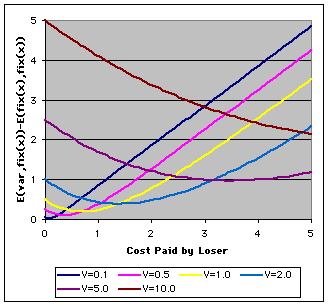Notice that as V gets larger the minimum difference between the two payoffsincreases. (If you are "Thomas from Missouri" and want me to showyou the low V graphs inmore detail, press here.)

So there you have it. For any cost paid by the winner, m, E(var,fix(x))> E(fix(x), fix(x)). So since this is true and sinceE(var, var) = E(fix(x), var), then var is evolutionarilystable against any fix(x)!

 Problems1. Write an expression for the lifetime cost to a var strategistof quitting at a cost of exactly x. Answer2. Write an expression for the lifetime cost to a var strategist forlosing contests where the winner was willing to pay m? Answer3. What is E(var, fix(0)) in the caseof a tie? Answer

Return to the "Contents"

Things to Remember Aboutthe 'Var' Strategy

Perhaps the most striking thing about the var strategy is thatits opponent never can know when it will quit. We have seen that the overallpattern of quitting is described by an exponential decay type of Poissondistribution with a rate constant equal to 1/V.Thus, an opponent can "learn" in generalterms what its var opponent would do. It could "know" thatit was most likely to quit early in a contest and that the chance of quittingper unit of contest display cost is exp(-1/V). From this, it is possibleto calculate (or learn from experience) the expected outcome of contestsof various costs.

However, even if it knew these things, it could never know whether ornot 'var' really would quit with the next increment of cost. Thus, no amountof experience with 'var' strategists will allow an opponent any edge overit.

The other thing to reiterate about var is that there is a logicto its quitting. It is tied to the resource value -- the greater that value,the less likely that var will quit at any particular cost and asa consequence it is potentially willing to accept a higher cost contest.Also, since 'var' always quits most frequently early in contests, the chancethat it will pay large costs relative to a resource value are low.

 "Are You Feeling Lucky,Punk?"In the classic Clint Eastwood thriller, Dirty Harry, the Eastwoodcharacter asks a naer-do-well to predict the future and guess whether ornot there bullets left in Eastwood's gun. So what do you think? Are youfeeling lucky1. The chance of getting killed in a scheduled commercial airline crashis roughly on the order of one in several million. It is about the samechance the earth has of being hit by a large meteor, small asteroid, orcomet. Discuss whether or not someone who flies commercial airlines daily(e.g., a flight attendant or pilot) for years is more likely on her or hisnext flight to be in a fatal accident. Likewise, the earth has notbeen hit by a really big one for about 65 million years. Are we more likelyto be hit now than we were say 60 million years ago (5 million after thelast one). Are you more likely to win on your next lottery entry (tax onstupidity) if you haven't won in the past and less likely if you have won?What does all of this have to do with the war of attrition? Discussion

Return to the "Contents"

#### Testing to see if Animals areUsing a 'Var-like' Strategy

There are a number of famous examples of animals that appear to be playingsimple waiting games. We will not go into them here because they are wellpresented both in the literature and in just about every animal behaviortext book. Perhaps the classic is the dung fly, Scatophaga stercoraria,studied heavy by Parker and Parker and Thompson (refs). The interested reader is urged to consult thesepapers or any number of behavioral ecology texts. We will finish this page,however, with the following question (which was addressed by Parker andThompson):

 ? Suppose that someone demonstrated that animal waitingtimes corresponded to those predicted by eq. 9 Does that constitute sufficient proof that a mixedESS described by eq. #9 exists? Explain.ANS

Return to the "Contents"

### Answers to Problems and Questions

Problems dealing with the calculationof P(m)

1. What is the cumulative chance of quitting between a cost of 0 andinfinity if V=1? V=5? V=0.5?

It makes no difference what the value of V is in this case. Any numberto the infinite power is infinite and the inverse of infinity is essentiallyzero. Therefore P(m) = 1.0 in all cases:

P(m)=1 - (1 / e^(infinity))=1 - (1 / infinity) = 1 - 0 = 1

2. What is the cumulative chance of quitting between a cost of 0 and0.6 if V=1? V=0.5?

For V=1: P(m = 0.6) = 1 - (1 / e^(0.6/0.5)) = 1 - (1 / e^(1.2) =

1 - (0.30) = 0.7

(return to previous placein text)

Questions About Chances ofContinuing

1. Name the probability distributions that we saw earlier that give(i) chances of continuing up to a certain cost or (ii) quitting as of acertain cost.

Ans: Q(m)and P(m),respectively

(return to previous place intext)

2. If eq. 11 gives the chance of continuing for a unit ofcost, write an expression that gives the chance of quitting per unit cost.

Ans: = 1 - exp(-1/V) -- recall that eq. 8 (chanceof quitting) is nothing more than 1 - eq. 9 (i.e., 1 - Q(m)). Now since eq. 9 is essentiallythe same as eq.11, then (1 - eq. 11) = (1 - exp(-1/V)) and this gives us the chanceof quitting.
So, for example, if V = 1, chance of quittingper unit cost is 0.632.

(return to previous place intext)

Questions About the Mixed Strategy Var

1. Compare what a contestant sees when it confrontsa population consisting entirely of 'var' strategists as compared to a populationthat is an equilibrial mix of pure supporting fix(x) strategies. Would thecontestant see any difference in these two situations?

Answer: No, they are equivalent. In both cases, the contestanthas no idea which maximum cost it is facing (provided that encounters withdifferent fix(x) supporting strategies are random in the mixed populationand that in neither case the maximum cost is tipped before being reached).

Return to previous place

2. How would you express the idea of constantrate of quitting with respect to a population of pure strategists who togetherproduce an equilibrium?

Answer: One way would be to say that in any contest with membersof this population, there is a constant chance per increment of cost thatone' s opponent will quit. This corresponds to the idea that one's chanceof opposing a given type of supporting strategist (maximum x) would be equalto its frequency in the population (as determined by integrating eq. #6). Supportingstrategies with low maximum x values would be more common so you would bemore likely to face them.

Return to previous place

3. Why is it crucial that no informationas to var's intention to continue or quit a contest be passed onto its opponent?

If the opponent has some reason to know var's intentions, therewill be strong selective pressure for it to act in a way that thwarts varand serves its own best interests. For instance, if it is certain that varwill not quit before reaching the opponents max cost, it will pay theopponent to quit immediately and cut its losses. Likewise, if varis certain to quit on the next move or over the next bit of cost, it willpay the opponent to wait var out and gain the resource (as comparedto var who in this case gains nothing).

(return to previous placein text)

4. How do you estimate the probabilitythat a var strategist will win a contest of cost x?

This is equal to Q(m) since Q(m) gives the chance thatvar has not quit as of cost x=m.

(return to previous placein text)

5. How do you estimate the probabilitythat a var strategist will lose a contest of cost x?

This is equal to P(m) since P(m) gives the cumulative chancethat var has already quit as of some cost x=m.

(return to previous placein text)

6. How do you estimate the probabilitythat a var strategist loses by paying a cost between x and x+dx?

This is equal to delta P(m) since delta P(m) gives thechance that var has endured to cost x=m without quitting butwill quit before paying cost x+dx (i.e., m+dm) wheredx or dm is some additional cost.

(return to previous placein text)

Calculation of the Chance of Var Paying a Specific Cost

7. Should the chance of a var quittingbetween 0.60 and 0.61 be greater or less than the chance of quitting between0.60 and 0.62? Explain.

It should be less for the smaller range of costs -- i.e., less in 0.60to 0.61 than in 0.60 to 0.62. In this case, all we have done is make a costinterval larger by 0.01. So, there are more quitting times in this largerinterval and therefore a greater total probability that an individual varwill quit within this interval.

(return to previous placein text)

8a. What is the chance of quitting withinthe specific cost interval of 0.60 and 0.61 if V=1? V=0.5?

for V=1: deltaP(m)=exp(-0.60 ) - exp(-0.61) = 0.00546

for V=0.5: deltaP(m)=exp(-0.60 / 0.5) - exp(-0.61 / 0.5) = 0.00596

(return to previous placein text)

8b. What is the cumulative chance of quittingwithin the specific cost interval of 1.0 and 1.01 if V=1? V=0.5? Comparethese answers with those you go in the last problem -- why is there a differencein probability even though delta m is the same (0.01) in both cases?

for V=1: deltaP(m)=exp(-1.0) - exp(-1.01) = 0.00366

for V=0.5: deltaP(m)=exp(-1.0 / 0.5) - exp(-1.01 / 0.5) = 0.00268

Notice that the chance of QUITTING WITHIN A SPECIFIC COST INTERVAL(delta P(m)) OF A CONSTANT RANGE (0.01) DECREASES AS THE AVERAGECOST OF THE INTERVAL INCREASES. This is not because the chance of quittingper 0.01 increment in cost has changed. Indeed, it is always proportionalto 1/V, regardless of the interval.

So why the difference? The difference reflects the lower chance thatan individual will actually have played to the higher cost. Thus, the chanceof actually having played to x = 0.60 is P(0.6) = 0.549 but the chance ofplaying all the way to x = 1.00 is P(1.00) = 0.368. If you apply a constantchance of remaining over the next 0.01x to each of these numbers (if V =1.0, it is 0.99) you will see that fewer actually quit in the second interval(because there are fewer there to quit!). There will be more about thisin the text.

(return to previous placein text)

Problems dealing with the calculationof costs to var in losses

1. Write an expression for the lifetime cost to a var strategistof quitting at a cost of exactly x.

Answer: This is given by p(x)dx and it is a very smallnumber.

Return to previous place intext

2. Write an expression for the lifetime cost to a var strategist forlosing contests where the winner was willing to pay m?

Var loses any contest that costs less than m. There are lots of waysthis can happen -- each losing cost has a unique probability of occurrencebased on 'var's probability density function. Thus:Return to previous place intext

3. What is E(var, fix(0)) in the case ofa tie?

Following our usual rule, each side wins 50% of the time. Since thereis a 100% chance that var will play at time 0 and the cost = 0, then E(var,fix(o))=0.5*{(V-m)-m}=0.5 * (V - 0) - 0 = 0.5V.

(return to previous placein text)

Note about the term "Learn":I use the term learn loosely -- it could mean "learn" in the usualsense of learning and memory or it may be that we are simply talking aboutmaking an appropriate evolutionary response -- selection for responses thatwork against a fixed wait time. In either case, an appropriate responsearises to a particular fixed strategy.

(return to previous place in text)

"Are You Feeling Lucky, Punk?"

In the classic Clint Eastwood thriller, Dirty Harry, the Eastwoodcharacter asks a naer-do-well to predict the future and guess whether ornot there bullets left in Eastwood's gun. So what do you think? Are youfeeling lucky?

1. The chance of getting killed in a scheduled commercial airlinecrash is roughly on the order of one in several million. About the samechance the earth has of being hit by a large meteor, small asteroid, orcomet. Discuss whether or not someone who flies commercial airlines daily(e.g., a flight attendant or pilot) for years is more likely on her or hisnext flight to be in a fatal accident. Likewise, the earth has notbeen hit by a really big one for about 65 million years. Are we more likelyto be hit now than we were say 60 million years ago (5 million after thelast one). Are you more likely to win on your next lottery entry (tax onstupidity) if you haven't won in the past and less likely if you have won?What does all of this have to do with the war of attrition?

All of these chances are independent. In these cases, there is a moreor less constant probability per flight of a disaster (this might be theworst example of the three since clearly a poor pilot, bad weather, poormaintenance or whatever could change your odds) -- what happens on otherflights does not affect the next one you get on. The same with asteroidsand lottery tickets. As with 'var', a constant probability means that itcan happen any time or maybe even not at all. The main difference betweenthese examples and the war of attrition is that in the 'war' we are concernedwith the distribution of quitting costs while in the other examples theemphasis is on the constant probability of some event.

Return to your previous placein the text

 Copyright © 1999 by Kenneth N. Prestwich College of the Holy Cross, Worcester, MA USA 01610 email: kprestwi@holycross.edu About Fair Use of these materials Last modified 12 - 1 - 09